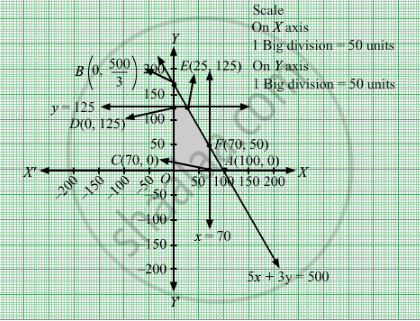# A Company Sells Two Different Products, a and B. the Two Products Are Produced in a Common Production Process, Which Has a Total Capacity of 500 Man-hours. - Mathematics

Sum

A company sells two different products, A and B. The two products are produced in a common production process, which has a total capacity of 500 man-hours. It takes 5 hours to produce a unit of A and 3 hours to produce a unit of B. The market has been surveyed and company officials feel that the maximum number of unit of A that can be sold is 70 and that for B is 125. If the profit is Rs 20 per unit for the product A and Rs 15 per unit for the product B, how many units of each product should be sold to maximize profit?

#### Solution

Let units of product A and units of product B were manufactured.
Clearly, $x \geq 0, y \geq 0$

It takes 5 hours to produce a unit of A and 3 hours to produce a unit of B.The two products are produced in a common production process, which has a total capacity of 500 man-hours.

$5x + 3y \leq 500$

The maximum number of unit of A that can be sold is 70 and that for B is 125.

$x \leq 70$
$y \leq 125$

If the profit is Rs 20 per unit for the product A and Rs 15 per unit for the product B. Therefore, profit units of product A and units of product B  is Rs 20x and Rs 15y respectively.

Total profit  = Z = $20x + 15y$

The mathematical formulation of the given problem is

Max Z = $20x + 15y$

subject to

$5x + 3y \leq 500$

$x \leq 70$

$y \leq 125$

$x \geq 0, y \geq 0$

First we will convert inequations into equations as follows:
5x + 3y = 500, x = 70, y = 125, x = 0 and y = 0

Region represented by 5x + 3y ≤ 500:
The line 5x + 3y = 500 meets the coordinate axes at A1(100, 0) and $B_1 \left( 0, \frac{500}{3} \right)$ respectively. By joining these points we obtain the line 5x + 3y = 500. Clearly (0,0) satisfies the 5x + 3y = 500. So, the region which contains the origin represents the solution set of the inequation 5x + 3y ≤ 500.
Region represented by x ​ ≤ 70:
The line ​x = 70 is the line passes through C1(70, 0) and is parallel to Y axis. The region to the left of the line x = 70 will satisfy the inequation x ​ ≤ 70.
Region represented by y ​ ≤ 125:
The line ​y = 125 is the line passes through D1(0, 125) and is parallel to X axis. The region below the  the line y = 125 will satisfy the inequation y ​ ≤ 125.
Region represented by ≥ 0 and y ≥ 0:
Since, every point in the first quadrant satisfies these inequations. So, the first quadrant is the region represented by the inequations x ≥ 0, and y ≥ 0.
The feasible region determined by the system of constraints 5x + 3y ≤ 500, x ​ ≤ 70, y ​ ≤ 125, x ≥ 0 and y ≥ 0 are as follows.The corner points are  O(0, 0), D1 $\left( 0, 125 \right)$ , E1(25, 125), F1(70, 50) and C1(70, 0).The values of Z at the corner points are

 Corner points Z = $20x + 15y$ O 0 D1 1875 E1 2375 F1 2150 C1 1400

The maximum value of Z is 2375 which is at E1
$\left( 25, 125 \right)$ .
Thus, maximum profit is Rs 2375, 25 units of A and 125 units of B should be manufactured.

Concept: Graphical Method of Solving Linear Programming Problems
Is there an error in this question or solution?

#### APPEARS IN

RD Sharma Class 12 Maths
Chapter 30 Linear programming
Exercise 30.4 | Q 33 | Page 54